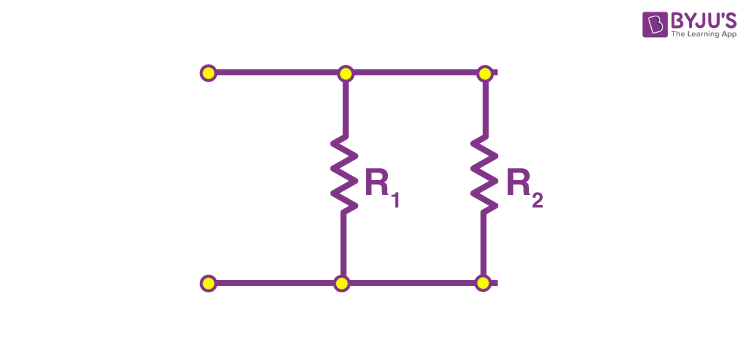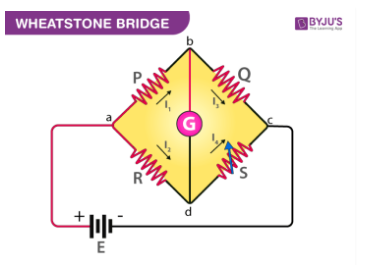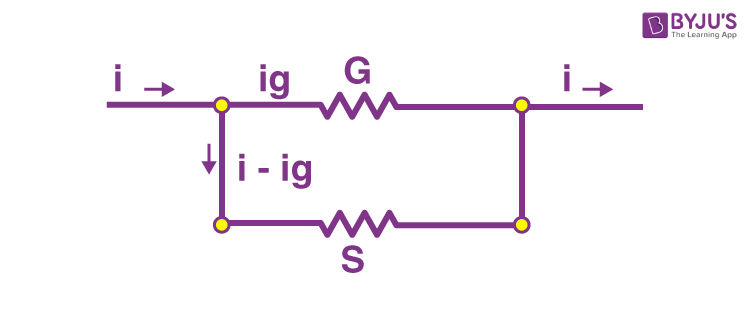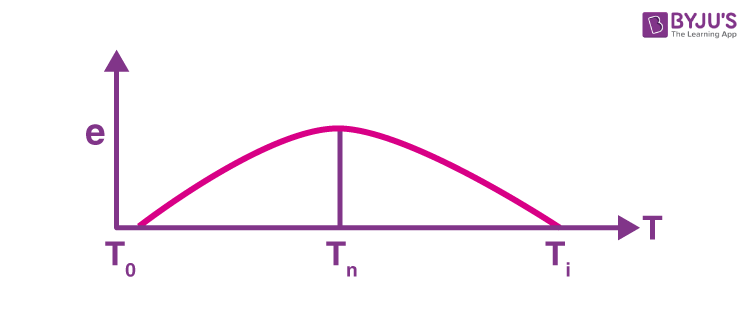# Important Current And Electricity Formulas For JEE

Electric current is the movement of charges through the wire. Current is measured in Amperes. For the charge to flow through the circuit, the circuit must be a closed circuit. In other words, the path from the power supply through the circuit, back to itself must be uninterrupted. The current flows through the circuit if there is a potential difference.

You can learn about electric current by following the attached link. Meanwhile, students can also check the important formulas for this topic below.

## Current Electricity Formulas

1. Current

I = neAVd

n is the number of free electrons per unit volume

A is the area of cross-section

e is the charge of the electron

Vd is the drift velocity

2. Current Density

j = i/A = σE

i is the current

A is the cross-sectional area

E is the electric density

σ is the conductivity

3. Drift speed

$V_{d}=\frac{1}{2}\frac{eE}{m}\tau =\frac{i}{neA}$

4. Resistance of the wire

R =ρl/A, where ρ=1/σ

ρ is the resistivity

σ is the conductivity

5. Temperature dependence of resistance

R=R0(1+ αΔT)

R0 is the original resistance

R is the resistance at temperature T

α is the temperature coefficient of resistance

6. Ohm’s law

V=IR

V is the voltage

I is the current

R is the resistance

7. Kirchhoff’s Law

(i) Junction Law: The algebraic sum of all the currents directed towards a node is zero.

i.e.,∑node Ii=0.

(ii) The Loop Law: The algebraic sum of all the potential difference along a closed loop in a circuit is zero.

i.e., ∑loop ΔVi=0.

8. Resistance in parallel1/Req = 1/R1 +1/R2

9. Resistance in seriesReq = R1 +R2

10. Wheatstone bridgeIn a balanced Wheatstone bridge

P/Q = R/S

11. Electric Power

P = V2/R=I2R =IV

12. Galvanometer as an AmmeterigG = (i – ig)S

G is the resistance of the galvanometer

S is the shunt resistance

i is the maximum current measured by the ammeter

ig is the maximum current passed through the galvanometer

13. Galvanometer as a VoltmeterV= ig(R+G)

G is the resistance of the galvanometer

Ig is the maximum deflection of galvanometer

R is the high resistance connected in series

14. Charging of capacitors

q(t) = CV(1- e-t/RC)

15. Discharging of capacitors

q(t) = q0e-t/RC

16. Time Constant in RC circuit

τ = RC

17. Peltier effect

Peltier effect (emf) = ΔH/ΔQ = Peltier heat/Charge transferred

18. Seeback effect(i) Thermo-emf : e=aT+(1/2)bT2

(ii) Thermoelectric power : de/dt=a+bT

(iii) Neutral temperature: Tn = -a/b

(iv) Inversion temperature : Ti= -2a/b

Tn is the neutral temperature

Ti is the inversion temperature

T0 is the temperature of the cold junction

a and b are the parameters of the materials used

19. Thomson effect

emf e = ΔH/ΔQ = Thomson heat/Charge transferred = σΔT

The mass deposited is

m= Zit = (1/F)Eit

Where, i is current, t is the time

Z is electrochemical equivalent

E is the chemical equivalent

F = 96485 C/g is Faraday constant.

Up Next: Important Gravitation Formulas for JEE

Test Your Knowledge On Important Current And Electricity Formulas For Jee!